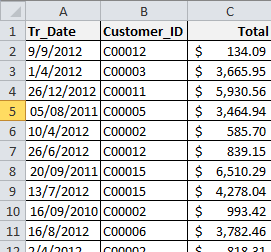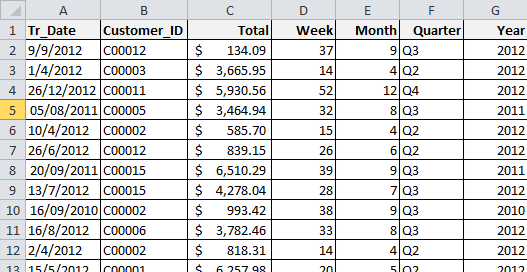# Excel Date Functions

As we all know by now, there are several functions in excel for handling date values. But they don’t seem much useful at first sight. That’s why I decided to post some daily applications for excel date functions.

A lot of times you will face data that doesn’t contains date values that are useful for your task at hand. In these kind of situations, you need to modify these data or create useful date values from them. And you will need excel date functions for this.

Lets have a quick look at most useful excel date functions:

I used TODAY() as variable for this list of formulas to introduce possibilities. You can use plain dates (like 08/05/2013), cell references (like A1) or other functions (like shown below) in your date functions.

There are certain number matches for all dates in excel. For example 08/05/2013 = 41402 . So you can also use this value in any date function too. It will give the same results as 08/05/2013.

Function                               Result

=NOW()                                 08/05/2013 20:03

=TODAY()                             08/05/2013

=TODAY()-1                          07/05/2013

=DAY(TODAY())                    8

=WEEKNUM(TODAY())       19

=WEEKDAY(TODAY())        4

=MONTH(TODAY())             5

=YEAR(TODAY())                2013

Like shown above, using these functions you can extract day, week, month, year from a date with ease. But what about quarters? If you need quarter info for your data, unfortunately you will need to create it yourself. Here is how it is done:

In order to find which quarter today belongs to, we can use this function:

=ROUNDUP(MONTH(TODAY())/3;0)

This small formula
will evaluate the month of any given date by dividing it to 3 and rounding the result upwards.

For Example: For A1 to have value 12/05/2013

=ROUNDUP(MONTH(A1)/3;0)

=ROUNDUP(5/3;0)

=ROUNDUP(1.66;0)

=2

Now it is time for some exercise:

We are going to generate different types of date information from transaction dates for weekly, monthly, quarterly and yearly analysis.

This is our sample data:We will turn it to this:Lets see the formulas used in this table. I’m going to give formulas for the first row, then you can drag them down till the end of the table.

Week:  =WEEKNUM(A2)

Month:  =MONTH(A2)

Quarter:   =ROUNDUP(MONTH(A2)/3;0)

Year:  =YEAR(A2)

I hope that this tutorial will be useful for you while dealing with dates in Excel.

For more formula/function tutorials you can visit our Formulas Category.

#### You may also like...

This site uses Akismet to reduce spam. Learn how your comment data is processed.